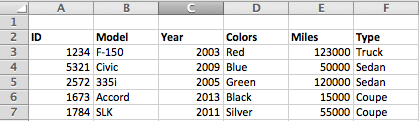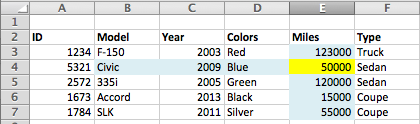A few weeks ago we covered INDEX MATCH as a replacement for VLOOKUP in excel. We only scratched the surface though. There are many more uses for INDEX, MATCH and VLOOKUP, using them together can even help you in a few tricky situations where a simple VLOOKUP or INDEX MATCH doesn’t do the trick.Using MATCH to Deal with Inserted Columns in VLOOKUP

One of the major issues with VLOOKUP is breaking the formula when you insert new columns. VLOOKUP uses a static index number to reference, if the order or number of columns changes it won’t reference the correct value and you’ll have to rewrite the function.

By using MATCH we can dynamically select columns in a range, effectively selecting the column by name rather than location. It’s pretty simple to implement. Instead of using the column index number, simply insert a match formula in the place of the column index number.

VLOOKUP(lookup_value, table_array, MATCH(), range_lookup)

Incase you need a refresher; MATCH is used to find the location of a value in an array. It looks something like this,

MATCH(lookup_value,lookup_array,match_type)

To simplify it, the look up value is what you are looking for, the lookup array is the row you want to search, and match type is used if you want to perform an exact match (which you usually do).

The function then looks for a value in the specified row and returns the location of the value. So if we select the header row, we can match the placement of the value, or for our purposes, the column name. This ends up being the same thing as the column index number.

VLOOKUP(lookup_value, table_array, MATCH(lookup_value, lookup_array, match_type), range_lookup)

Using INDEX MATCH MATCH to Find Values in a Matrix

We just found a way to boost VLOOKUP’s usefulness. What if you could also search in two dimensions, by both row and column, using two dynamic values?

That is exactly what INDEX MATCH MATCH does; it uses a row and column value and returns the intersection of the specified row and column.

INDEX(array_row,row_num,column_num)

Earlier we covered that the MATCH function returns the index of an array. So if we use MATCH in place of the row_num and col_num we should be able to get both of those values pretty easily.

If it helps, you can think of the formula as,

INDEX(The Table You’re Searching, The Row You Want, The Column Holding the Value)

Walk ThroughAbove is a table with a selection of cars in stock at a local dealer. I need to pull numbers easily without having to go through searching the entire table. For the example we’ll just have to pretend the table is much larger, making it harder to ready completely.

Using our example data, I want to know the mileage of the Civic in stock. To find this I can break it down into parts.

First we need to select the table,

INDEX(B2:F7,row_num, col_num)

Next we need to select the row. Since I’m looking for data on the Civic, I search for that value in the model column.

I can find that value by using,

MATCH(“Civic”,B2:B7,0)

Now I insert it into our INDEX function.

INDEX(B2:F7, MATCH(“Civic”B2:B7,0), col_num)

Now to get the column number, I do the same thing but I select the top level rows. To find the miles I use,

MATCH(“Miles”,B2:F2,0)

Insert it into the INDEX function and you end up with

INDEX(B2:F7, MATCH(“Civic”, B2:B7,0), MATCH(“Miles”,B2:F2,0))Which returns the intersection of “Civic” and “Miles”, giving us a value of 50,000.

Showing off the functions flexibility, to find the F-150’s miles, I simply change the first argument of the first MATCH function to “F-150”. If I want to find the year of the Civic, I only have to change “miles” to “year” in the second MATCH function.

Highlighting the utility of INDEX MATCH, if I know the model I want and I need the ID number, which is to the right of the column, I can simply change the second MATCH function to MATCH(“ID”,A2:A7,0). Using INDEX MATCH lets me pull the ID number without restructuring the table like I would need to for VLOOKUP.

While these might not come in handy everyday, I’ve found them to be extremely useful when needed. It takes a little bit of effort to learn but when a problem presents itself you will wonder how you got by before without these.